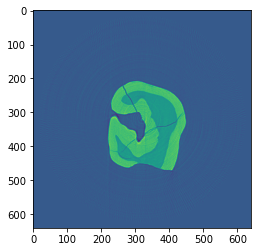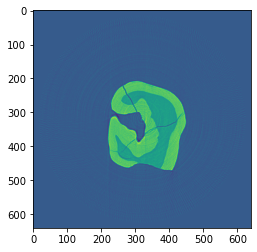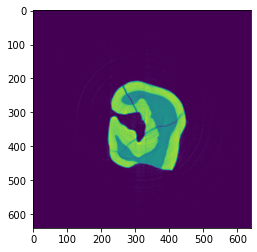# TomoPy with the ASTRA toolbox¶

Here is an example on how to use the ASTRA toolbox through its integration with TomoPy as published in [A2].

To reconstruct the image with the ASTRA toolbox instead of TomoPy, change the algorithm keyword to tomopy.astra. Specify which projection kernel to use (proj_type) and which ASTRA algorithm to reconstruct with (method) in the options keyword.

These two cells are an abbreviated setup for Reconstruction with TomoPy.

:

import dxchange
import matplotlib.pyplot as plt
import tomopy

:

proj, flat, dark, theta = dxchange.read_aps_32id(
fname='../../../source/tomopy/data/tooth.h5',
sino=(0, 2),
)
proj = tomopy.normalize(proj, flat, dark)
rot_center = 296
proj = tomopy.minus_log(proj)


For example, to use a line-based CPU kernel and the FBP method, use:

:

options = {'proj_type': 'linear', 'method': 'FBP'}
recon = tomopy.recon(proj,
theta,
center=rot_center,
algorithm=tomopy.astra,
options=options,
ncore=1)
plt.imshow(recon[0, :, :])
plt.show()

Reconstructing 1 slice groups with 1 master threads...If you have a CUDA-capable NVIDIA GPU, reconstruction times can be greatly reduced by using GPU-based algorithms of the ASTRA toolbox, especially for iterative reconstruction methods.

To use the GPU, change the proj_type option to 'cuda', and use CUDA-specific algorithms (e.g. 'FBP_CUDA' for FBP):

:

options = {'proj_type': 'cuda', 'method': 'FBP_CUDA'}
recon = tomopy.recon(proj,
theta,
center=rot_center,
algorithm=tomopy.astra,
options=options,
ncore=1)
plt.imshow(recon[0, :, :])
plt.show()

Reconstructing 1 slice groups with 1 master threads...Many algorithms of the ASTRA toolbox support additional options, which can be found in the documentation. These options can be specified using the extra_options keyword.

For example, to use the GPU-based iterative SIRT method with a non-negativity constraint, use:

:

extra_options = {'MinConstraint': 0}
options = {
'proj_type': 'cuda',
'method': 'SIRT_CUDA',
'num_iter': 200,
'extra_options': extra_options
}
recon = tomopy.recon(proj,
theta,
center=rot_center,
algorithm=tomopy.astra,
options=options,
ncore=1)

Reconstructing 1 slice groups with 1 master threads...